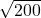## There were 3450 previously owned homes sold in eastern Washington in the year 2010. The distribution of the sales prices of these homes was

Question

There were 3450 previously owned homes sold in eastern Washington in the year 2010. The distribution of the sales prices of these homes was as strongly right-skewed with a mean of $406,274 and a standard deviation of$49,981. If all possible simple random samples of size 200 are drawn from this population and the mean is computed for each of these samples which of the following describes the sampling distribution of the sample mean?

a. Right skewed with mean of $406,274 and standard deviation of$49,981.
b. Approximately normal with mean $406,274 and standard deviation$249
c. Approximately normal with mean of $406,274 and standard deviation of$49,981.
d. Right skewed with mean of $406,274 and standard deviation$3534
e. Approximately normal with mean of $406,274 and standard deviation$3534

in progress 0
2 weeks 2021-08-30T03:44:34+00:00 1 Answers 0 views 0

e. Approximately normal with mean of $406,274 and standard deviation$3534
Given,The distribution of the sales prices of these homes was as strongly right-skewed with a mean of $406,274 and a standard deviation of$49,981 & Sample of Size 200 Are Drawn .
Now, By the Central Limit Theorem, the approximately normal Mean is $406,274 . And, Standard deviation ⇒ s= σ / √n ⇒$49981 /⇒ $49981 / 10×√2 ⇒$4998.1 / √2 ⇔ $3534.72 ≈$3534